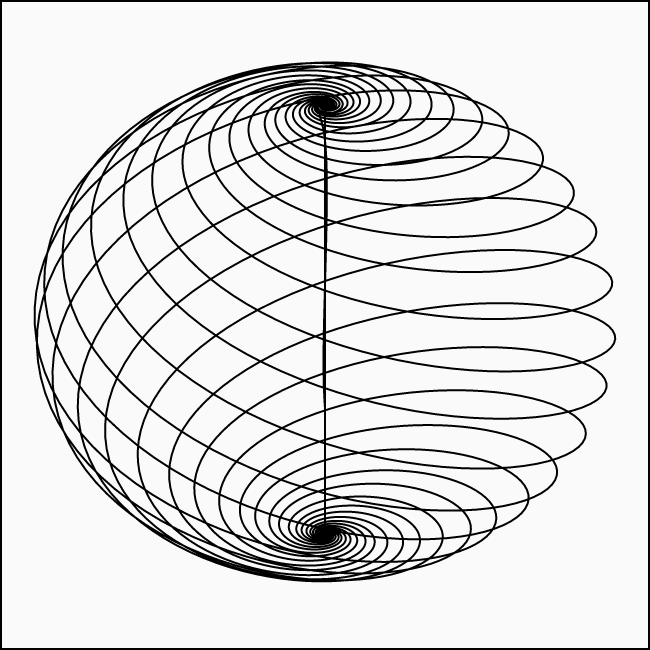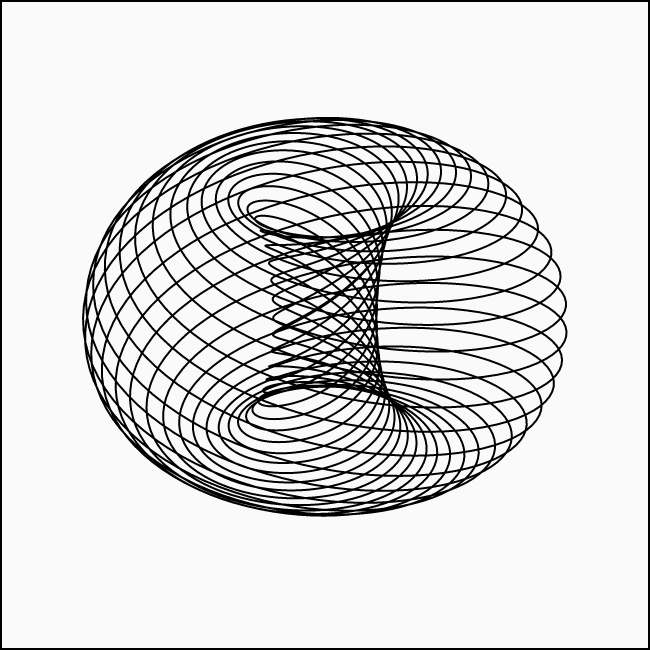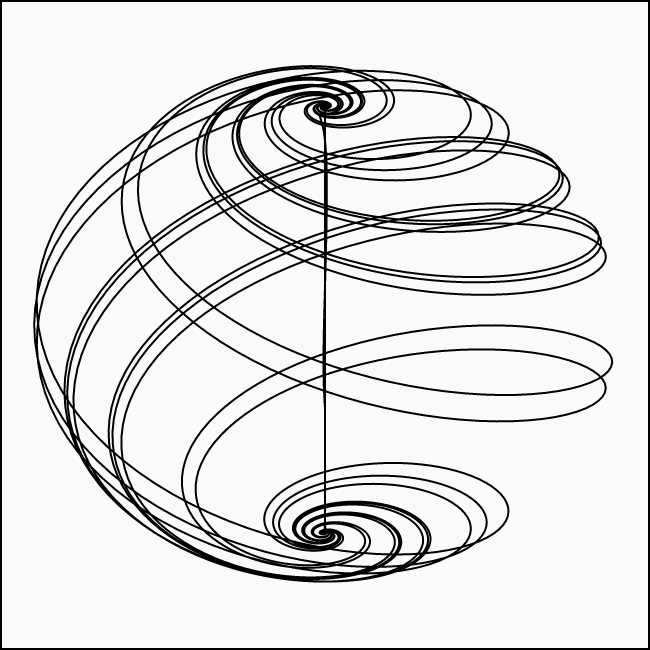# Langford Attractor

This attractor1 was found by William Finlay Langford in 1984.2 As the title of his paper suggests, the attractor is a bifurcation of a torus. What does that mean? Well, what he has done is taking a system of differential equations that resulted in a torus shape and simply added a bifurcation term to one of them. Jokes aside, he has probably done a lot more work to get to that point but looking at the equations and tinkering with the parameters let you imagine just that. Specifically, this term is $$\varepsilon\, z\, x^3$$ and it is added to $$\dot{z}$$. If you leave it out by setting $$\varepsilon = 0$$, you get a spiraly donut shape3:The inner tube is very thin here but when decreasing $$\alpha$$ to something like $$0.65$$, the shape becomes obvious:Now when increasing the bifurcation parameter (and keeping the original value for $$\alpha$$), the chaos begins. Here is it with $$\varepsilon = 0.001$$ and as you can see, this very slight change pushes the system out of symmetry:## Renders

Differential system:

$\dot{x} = x\, (z - \beta) - \omega\, y$ $\dot{y} = y\, (z - \beta) + \omega\, x$ $\dot{z} = \lambda + \alpha\, z - \frac{z^3}{3} - (1 + \varrho\, z)\, (x^2 + y^2) + \varepsilon\, z\, x^3$

Constants:

$\alpha = 0.95$ $\beta = 0.7$ $\lambda = 0.6$ $\omega = 3.5$ $\varrho = 0.25$ $\varepsilon = 0.1$1. Most online resources refer to this attractor as the “Aizawa Attractor” despite that Yoji Aizawa (it apparently got named after) seems to have nothing to do with it.4 How this confusion arose is unclear.

2. W.F. Langford, 1984. "Numerical Studies of Torus Bifurcations". In: T. Küpper, H.D. Mittelmann, H. Weber (eds), "Numerical Methods for Bifurcation Problems". International Series of Numerical Mathematics, Vol 70. doi:10.1007/978-3-0348-6256-1_19

3. This and the other simple images were generated using Processing, similar to this one I’ve done for the Lorenz Attractor

4. This was pointed out by: E. Fleurantin, J.D. Mireles James, 2019. "Resonant tori, transport barriers, and chaos in a vector field with a Neimark-Sacker bifurcation". Department of Mathematics, Florida Atlantic University.

🕸 💍